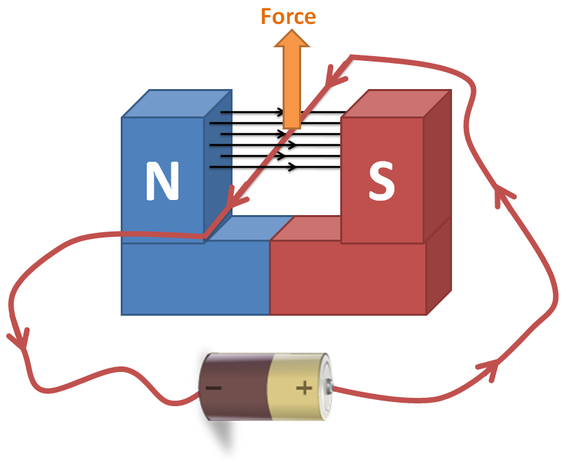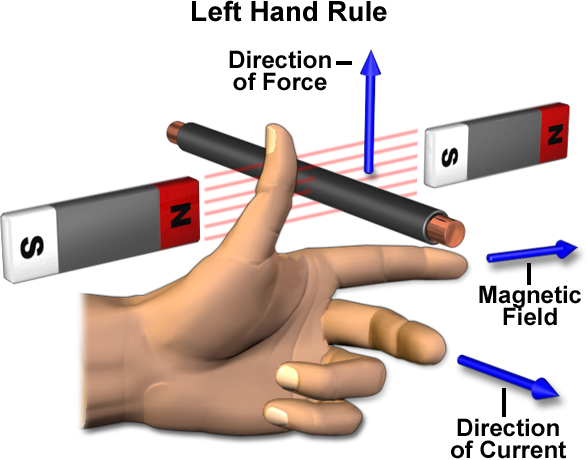# Fleming's Left Hand Rule

## The Motor Effect

The _motor effect ___occurs when a conductor carrying a current is placed in a magnetic field that is caused by a secondary magnet, the magnet and the conductor exert a force on each other.## Fleming’s Left Hand Rule

Fleming came up with a way of showing the orientation of force, current and magnetic field in relation to each other. It requires the use of your left hand.

Ensure that you know what the fingers and thumb represent.## Magnetic Force

Magnetic force can be calculated via this equation which is given in your exam:

Force = Magnetic flux density x current x length of wire

F = B x I x l

F = Force, Newtons (N)

B = Magnetic flux density, Tesla (T)

I = Current, Amps (A)

l = length of wire in the magnetic field, metres (m)

What is the effect called when a force is produced from placing a conductor inside an external magnetic field?